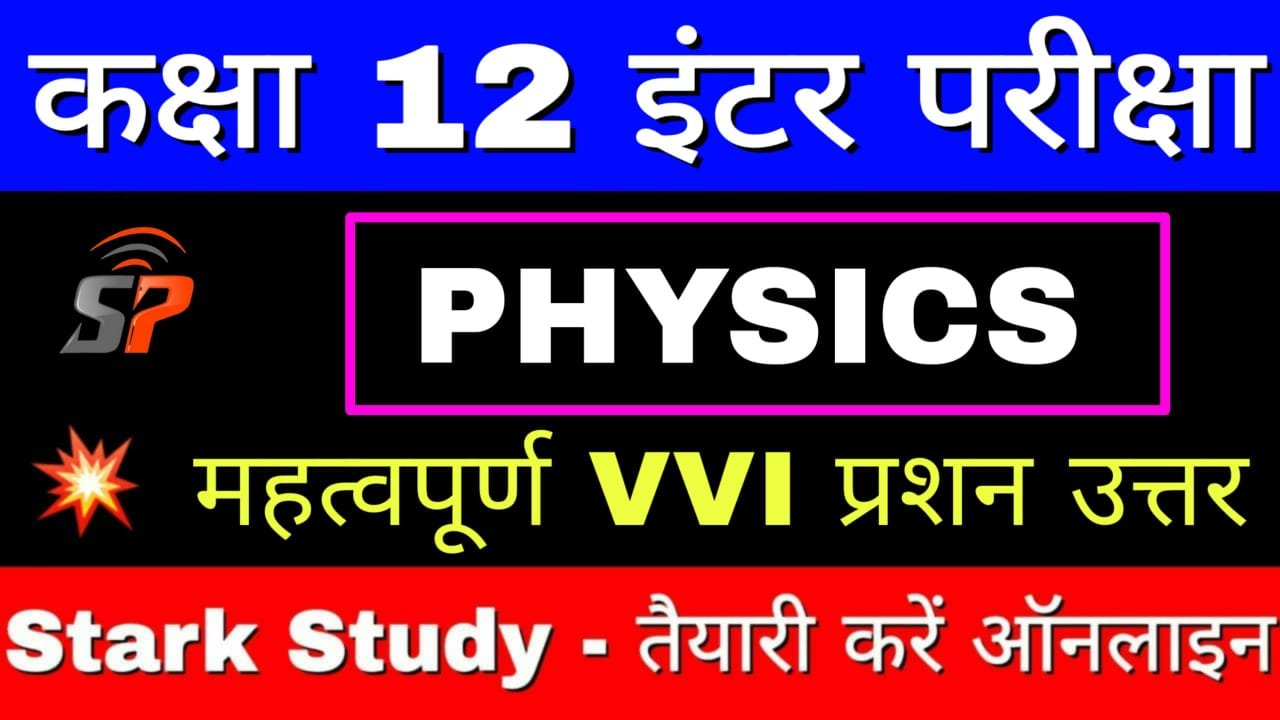12th Exam Physics VVI Objective Atoms Chapter Important Question

ATOMS

1. Cathode rays are :

(A) electrons

(B) neutrons

(C) protons

(D) photons

2. γ-rays are similar to :

(A) C-rays

(B) B-rays

(C) cathode rays

(D) X-rays

3. β-rays are fast moving :

(A) photons

(B) protons

(C) electrons

(D) neutrons

4. Which of the following series of hydrogen spectrum is in visible range ?

(A) Lyman series

(B) Balmer se

(C) Paaschen series

(D) Brackett series.

5. Electron-volt (eV) is the measure of:

(A) charge

(B) potential difference

(C) current

(D) energy

6. The specific charge of electron is :

(A) 1.8 x 1011 c/kg

(B) 1.8 x 10-19 c/kg

(C) 1.9 x 10-19 c/kg

(D) None of these

7. The number of neutrons in an atom of 90Th230 is :

(A) 90

(B) 140

(C) 230

(D) 320

8. The first model of atom in 1898 was proposed by :

(A) Ernst Rutherford

(B) Albert Einstein

(C) J. J. Thomson

(D) Niels Bohr

9. Rutherford’s experiments suggested that the size of the nucleus is about :

(A) 10-14 m to 10-12 m

(B) 10-15 m to 10-13 m

(C) 10-15 m to 10-14 m

(D) 10-15 m to 10-12 m

10. The Bohr model of atoms :

(A) assumes that the angular momentum of electrons is quantized

(B) uses Einstein’s photoelectric equation

(C) predicts continuous emission spectra for atoms

(D) predicts the same emission spectra for all types of atoms

11. Who explained the splitting of special lines in magnetic field ?

(A) Zeeman

(B) Bohr

(C) Summerfield

(D) Einstein

12. The mass of a neutron is :

(A) 1.00866 u

(B) 1.0866 u

(C) 1.866 u

(D) 0.1866 u

13. Half-life of a substance depends on:

(A) pressure

(B) temperature

(C) density

(D) None of these

14. The concept of electron spin was introduced by :.

(A) Becquerel

(B) Goudsmit

(C) Millikan

(D) Uhlenbeck and Goudsmit

15. β-decay produces :

(A) isobars

(B) isotopes

(C) isotones

(D) All the above

16. Transmutation of nuclei was discovered by :

(A) Rutherford

(B) Becquerel

(C) Pauli

(D) None of these

17. The energy equivalent to mass defect is called :

(A) binding energy

(B) internal energy

(C) external energy

(R) enthalpy

18. The binding energy of He is about :

(A) 28.3 eV

(B) 28.3 Mev

(C) 28.3 J

(D) 2.83 Mev

19. The binding energy of a deuteron is about :

(A) 2.22 MeV

(B) 2.22 J

(C) 2.22 eV

(D) None of these

20. Who discovered the positron ?

(B) Bohr

(C) Maxwell

(D) Anderson

21. The mass of proton is about :

(A) 9.11 x 10-31 kg

(B) 9.11 x 10-30 kg .

(C) 1.66 x 10-27 kg

(D) 1 kg

22. Chadwick was awarded the Nobel Prize in Physics in 1935 for his discovery of :

(A) electron

(B) proton

(C) neutron

(D) None of these

23. The half life of a radioactive decay is n times its mean life, n is equal to :

(A) 0.6930

(B) 0.0693

(C) 1 / 0.6930

(D) 0.3070

24. Rutherford is the unit of:

(B) energy

(C) photoelectric current

(D) magnetic field

25. The wavelength of Y -rays is of the order of:

(A) 10-8 m

(B) 10-5 me

(C) 10-11 m

(D) 10-22 m

26. Which series of hydrogen spectrum does not lie in infrared region?

(A) Humphreys series

(B) Pfund series

(C) Bracket series

(D) Lyman series

27. The energy of electron in first Bohr orbit of hydrogen atom is -13.6 eV. What is energy of electron in its 2nd Both-orbit.

(A) – 3.4 eV

(B) – 6.8 eV

(C) – 27.2 eV,

(D) + 3.4 eV

28. Full wave rectifier uses :

(A) two diodes

(B) three diodes

(C) four diodes

(D) five diodes

29. The atomic number and mass number for a specimen are Z and A respectively. The number of neutrons in the atom will be :

(A) A

(B) Z

(C) A + Z

(D) A-Z